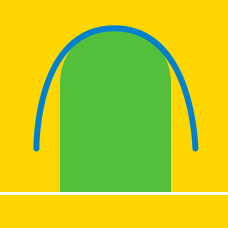Calculus

# Area between 2 curves

What is the area of the region bounded by the two curves $y= -{x}^2+2x+13, y= {x}^2 + 9 ?$

If $m$ is a positive real number such that the area of the region bounded by the curve $y={x}^2-x$ and the line $y=mx$ is $\frac{32}{3},$ what is $m?$

Compute the area bounded by the curve $y = x^3 - 6x^2 + 9x$ and the line $y = x$.

What is the area of the region bounded by the curves $y= {e}^x - 1, y= 4e^{-x} - 1, y=0?$

What is the area of the region bounded by the curve $y= -{x}^2+6x$ and the line $y=-8x ?$

×